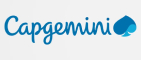New update is available. Click here to update.
Topics

# Rearrange String

Easy0/40
Average time to solve is 15m## Problem statement

You are given a string of lowercase characters. Your task is to rearrange (reorder) the string in such a way that no two adjacent characters are the same.

You have to return the rearranged string. If there exists more than one solution you can return any of them.If there is no such string you have to return “NO SOLUTION”. If your returned value is correct the program will print ‘CORRECT’ else ‘INCORRECT’.

For example :

``````If we are given a string "aabb", then the possible solutions are:

(i) abab
(ii) baba

We can see no two adjacent characters are the same in both strings.

So both (i) and (ii) are valid solutions.
``````
Detailed explanation ( Input/output format, Notes, Images )
Constraints:
``````1 <= T <= 100
1 <= N <= 10^4

Where ‘N’ is the length of the string.

Time limit: 1 sec
``````

#### Sample Input 1:

``````3
aac
azzz
abbc
``````

#### Sample Output 1:

``````aca
NO SOLUTION
abcb
``````

#### Explanation for Sample Output 1:

``````In test case 1, there exists only one solution which is ‘aca’. We can see no two adjacent characters are the same.

In test case 2, For the second string there exists no solution.

In test case 3, there exists more than one solution out of which ‘abcb’ is also a possible solution.
``````

#### Sample Input 2:

``````3
bbb
xyz
yaxx
``````

#### Sample Output 2:

``````NO SOLUTION
xyz
axyx
``````

#### Explanation for Sample Output 2:

``````In test case 1, there exists no solution.

In test case 2, there exists more than one solution out of which ‘xyz’ is a possible solution.

In test case 3, there exists more than one solution out of which ‘axyx’ is a possible solution.
``````Console